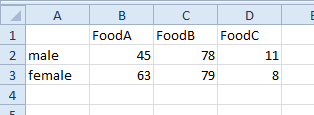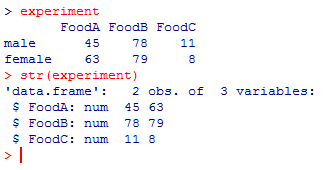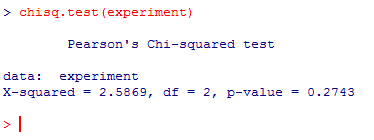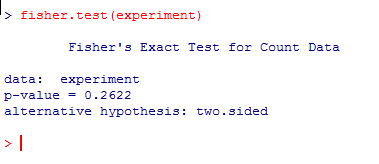# Comparing two variables – Chi-square test and Fisher’s exact test

The Chi-square test for independence (a.k.a. χ-square test or Pearson’s chi-square test of association) comes in handy when you need to compare two categorical variables and when the dataset is made of counts (whole numbers). Often this dataset will look like a “contingency table“, something like this:

 Food A Food B Food C male count 1A count 1B count 1C female count 2A count 2B count 2C

Of course, the nature of these variables will vary. Sometimes there will be only 2 “contingencies” per variable and your dataset will be limited to a 2×2 table, sometimes one or both of the variables will have many more contingencies and the complexity of your dataset will increase accordingly.

Regardless of the number of rows, columns and cells, the goal of the test is often one of these two:

• to define whether there is a link/association/dependence between the 2 variables.
• to define whether the outcome of an experiment follows a principle, a rule and thus match some expectations (goodness of fit).

Note: the result of the Chi-square test might be unreliable if the sample is small (below 10, some say below 5…). One may thus use Fisher’s exact test instead for such small samples. Anyway, Fisher’s exact test appears to be valid for all sample sizes.

Lets take an example. We test 3 different types of food (A, B and C) on male and female dogs and note the preference of each individual. We want to know whether there is a food preference that depends on gender. Let’s look at the data:Let’s create “manually” the dataframe that contains these data. First we load 2 vectors with the data for `male` and `female `(see line 1 and line 2 in the following code). Then we create the matrix `experiment` by combining these 2 vectors (line 3) and finally we give names to each column (line 4)

```male<-c(45,78,11)
female<-c(63,79,8)
experiment<-as.data.frame(rbind(male, female))
names(experiment)<-c("FoodA", "FoodB", "FoodC")
```

Before running the test, let’s check that the dataframe is correctly loaded by displaying it (line 1) and checking its structure (line 2) with `str()`:

```experiment
str(experiment)
```As shown above, the structure is indeed a dataframe with 2×3 entries, as expected. The dataset is now loaded. Let’s proceed with the Chi-square test (where the null hypothesis H0 is that food preference is independent of gender) for which the function is `chisq.test()` :

```chisq.test(experiment)
```The obtained p-value is above 0.05. The null hypothesis H0 is thus accepted: there is no gender-dependent food preference.

Should you have a preference for Fisher’s exact test, the function is  `fisher.test():`and the conclusion is the same as for the Chi-square test, in the present case.

Fant du det du lette etter? Did you find this helpful?
[Average: 0]